# CLASS 10 MATH NCERT SOLUTIONS FOR CHAPTER – 3 PAIR OF LINEAR EQUATIONS IN TWO VARIABLES EX – 3.2

## Pair of Linear Equations in Two Variables

Question 1.
Form the pair of linear equations of the following problems and find their solutions graphically:

(i) 10 students of class X took part in a Mathematics quiz. If the number of girls is 4 more than the number of boys, find the number of boys and girls who took part in the quiz.

(ii) 5 pencils and 7 pens together cost ₹50, whereas 7 pencils and 5 pens together cost ₹46. Find the cost of one pencil and that of one pen.

Solution:

(i) Let the number of boys and girls who took part in the quiz be x and y respectively.
Then according to the question: we have:
x + y = 10       …(i)
and y = x + 4   …(ii)
For graphical representation:
From equation (i), we have: y = 10 – x
When x = 2, then y = 10 – 2 = 8
When x = 5, then y = 10 – 5 = 5
When x = 3, then y= 10 -3 = 7
Thus, we have the following table of solution: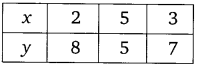From equation (ii), we have:     y =   x + 4
When x = 2, then y     = 2 +  4   = 6
When x = 3, then y       = 3 +  4   = 7
When x = 4, then y     = 4 +  4   = 8
Thus, we have the following table of solutions:Plotting the points of each table of solutions, we obtain the graphs of two lines, which intersect at a point P(3, 7). Therefore, the solution is x = 3 and y = 7.
Thus, the number of boys = 3 and the(ii) Let the cost of one pencil be ₹x
and the cost of one pen be ₹y
Then according to the question, we have:
5x + 7y = 50         …(i)
and 7x + 5y = 46      …(ii)
For graphical representation: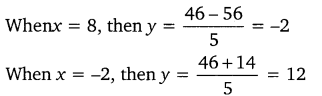Thus, we have the following table of solutions:Plotting the points of each table of solutions, we obtain the graphs of two lines intersecting at a point P(3, 5). Therefore, the solution is x = 3 and y = 5.
Thus, the cost of one pencil is ₹3 and that of one pen is ₹5.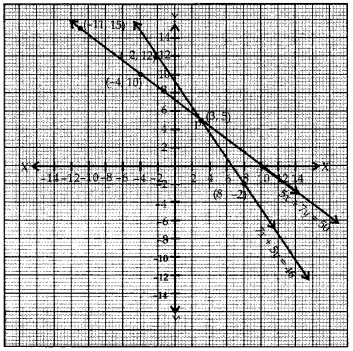Question 2.
On comparing the ratios$\frac { { a }_{ 1 } }{ { a }_{ 2 } }$$\frac { { b }_{ 1 } }{ { b }_{ 2 } }$ and$\frac { { c }_{ 1 } }{ { c }_{ 2 } }$ , find out whether the lines representing the following pairs of linear equations intersect at a point, are parallel or coincident:

(i) 5x – 4y + 8 = 0, 7x + 6y – 9 = 0
(ii) 9x + 3y + 12 = 0, 18x + 6y + 24 = 0
(iii) 6x – 3y + 10 = 0, 2x -y + 9 = 0

Solution: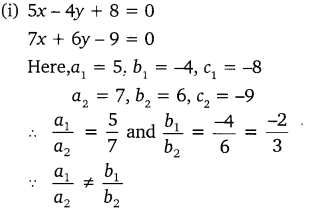Hence, the lines intersect at a point.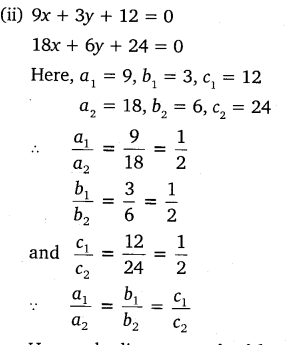Hence, the lines are coincident,Hence, the pair of linear equations has no solution, i.e., the lines are parallel.

Question 3.
On comparing the ratios$\frac { { a }_{ 1 } }{ { a }_{ 2 } }$$\frac { { b }_{ 1 } }{ { b }_{ 2 } }$ and$\frac { { c }_{ 1 } }{ { c }_{ 2 } }$, find out whether the following pairs of linear equations are consistent, or inconsistent:Solution:

(i) 3x + 2y – 5 = 0
2x – 3y – 7 = 0
Here, a= 3, b1 = 2, c1 = -5
a2= 2, b2 = -3, c2 = -7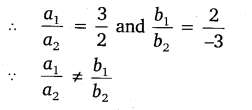Hence, this pair of linear equations is consistent.Hence, this pair of linear equations is inconsistent.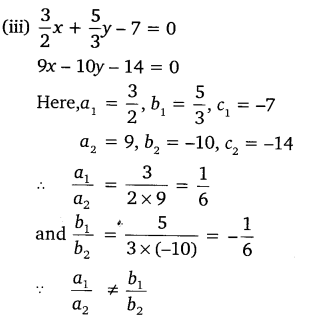Hence, this pair of linear equations is consistent.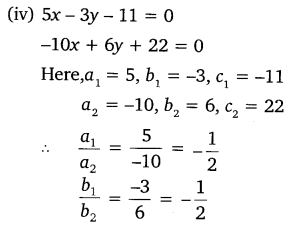Hence, this pair of linear equations is consistent.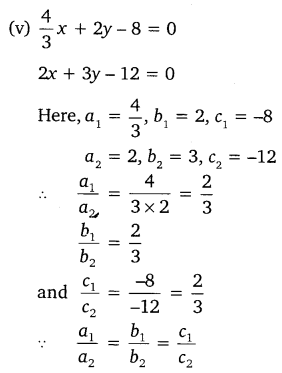Hence, this pair of linear equations is consistent

Question 4.
Which of the following pairs of linear equations are consistent/inconsistent? If consistent, obtain the solution graphically.

(i) x + y = 5, 2x + 2y = 10
(ii) x-y – 8, 3x – 3y = 16
(iii) 2x + y – 6 = 0, 4x – 2y – 4 = 0
(iv) 2x – 2y – 2 = 0, 4x – 4y – 5 = 0

Solution:

(i) x + y – 5, 2x + 2y = 10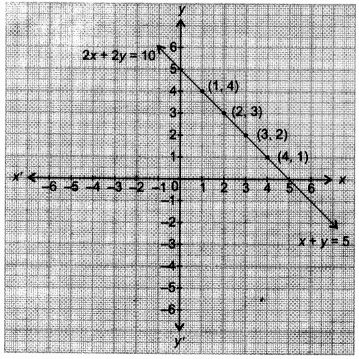Here,∴ System of equations is consistent and the graph gr represents coincident lines.
Table for equation (i),Table for equation (ii),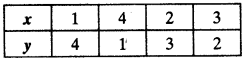(ii)
x – y = 8, 3x – 3y = 16
Here$\frac { a_{ 1 } }{ a_{ 2 } } =\frac { 1 }{ 3 } ,$Pair of equations is inconsistent. Hence, lines are parallel and(iii)
2x + y – 6 = 0, 4x – 2y – 4 = 0Pair of equations is consistent.
Table for equation 2x + y – 6 = ()Table for equation 4x – 2y – 4 = 0

(iv)
2x – 2y – 2 = 0, 4x – 4y – 5 = 0∴ Pair of equations is inconsistent. Hence, lines are parallel and system has no solution.

Question 5.
Half the perimeter of a rectangular garden, whose length is 4 m more than its width is 36 m. Find the dimensions of the garden graphically.

Solution:
Let the length of the garden be x m and its breadth be y m.
Then according to the question, we have:
x = y + 4
⇒  x-y = 4  …(i)
and x+y = 36    …(ii)
For graphical representation:
From equation (i), we have: y = x- 4
When x = 10, then y = 10 – 4 = 6
When x = 12, then y -12-4 = 8
When x = 20, then y = 20 – 4 = 16
Thus, we have the following table of solutions: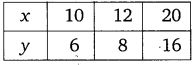From equation (ii), we have: y = 36
When x = 20, then y = 36 – 20 = 16
When x – 24, then y = 36 – 24 = 12
When x = 12, then y = 36 – 12 = 24
Thus, we have the following table of solutions: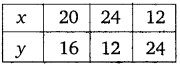Plotting the points of each table of solutions, we obtain the graphs of two lines intersecting at P(20, 16).
Therefore, the solution is x = 20 and y = 16.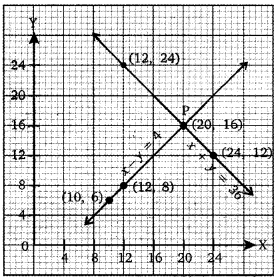Thus, the length of the garden is 20 m and its breadth is 16 m.

Question 6.
Given the linear equation 2x + 3y – 8 = 0, write another linear equation in two variables such that the geometrical representation of the pair so formed is:

(i) intersecting lines
(ii) parallel lines
(iii) coincident lines

Solution:
Given equation is 2x + 3y – 8 – 0
We have 2x + 3y = 8
Let required equation be ax + by = c

Condition :

(i) For intersecting lines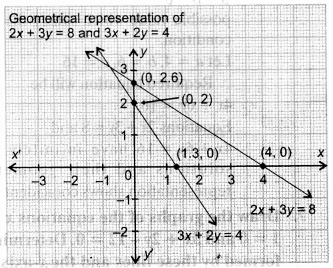$\frac { 2 }{ a } \neq \frac { 3 }{ b } \neq \frac { 8 }{ c }$ where a, b, c can have any value which satisfy the above condition.
Let a = 3, b = 2, c = 4
so,$\frac { 2 }{ 3 } \neq \frac { 3 }{ 2 } \neq \frac { 8 }{ 4 }$
∴ Equations are 2v + 3y = 8 and 3A + 2y = 4 have unique solution and their geometrical representation shows intersecting lines.

(ii) Given equation is 2x + 3y = 8 Required equation be ax + by = c

Condition :
For parallel lines$\frac { 2 }{ a } \neq \frac { 3 }{ b } \neq \frac { 8 }{ c }$
where a, b, c can have any value which satisfy the above condition.
Let, a = 2, b = 3, c = 4
Required equation will be 2x + 3y = 4Equations 2x + 3y = 8 and 2x + 3y = 4 have no solution and their geometrical representation shows parallel lines.

(iii)
For coincident lines: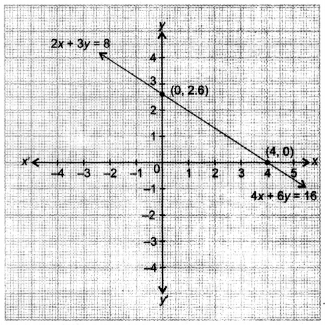Given equation is 2x + 3y = 8 Let required equation be ax + by = c For coincident lines.$\frac { 2 }{ 3 } =\frac { 3 }{ 2 } =\frac { 8 }{ 4 }$ where a, b, c can have any a b c possible value which satisfy the above condition.
Let a = 4, b = 6, c = 16 Required equation will be 4x + 6y = 16.
Equations 2x + 3y = 8 and 4x + 6y = 16 have infinitely many solutions and their geometrical representation shows coincident lines.

Question 7.
Draw the, graphs of the equations x – y + 1 = 0 and 3x + 2y – 12 = 0. Determine the coordinates of the vertices of the triangle formed by these lines and the x-axis, and shade the triangular region.

Solution:
Given:
x – y +1 = 0      … (i)
3x + 2y – 12 = 0    … (ii)
From equation (i), we have:
y = x+ 1
When x = 0, then y = 0 + 1 = 1
When x = 1, then y =1 + 1 = 2
When x = 3, then y = 3 + 1 = 4
When x = -1, then y = -1 + 1 = 0
Thus, we have the following table of solutions:Plotting the points on a graph paper, we get the shaded triangle ABC with vertices A(2, 3), B(-l, 0) and C(4, 0).#### CLASS 10 MATH NCERT SOLUTIONS FOR CHAPTER – 3 PAIR OF LINEAR EQUATIONS IN TWO VARIABLES EX – 3.3

Lorem ipsum dolor sit amet, consectetur adipiscing elit. Phasellus cursus rutrum est nec suscipit. Ut et ultrices nisi. Vivamus id nisl ligula. Nulla sed iaculis ipsum.

Company Name